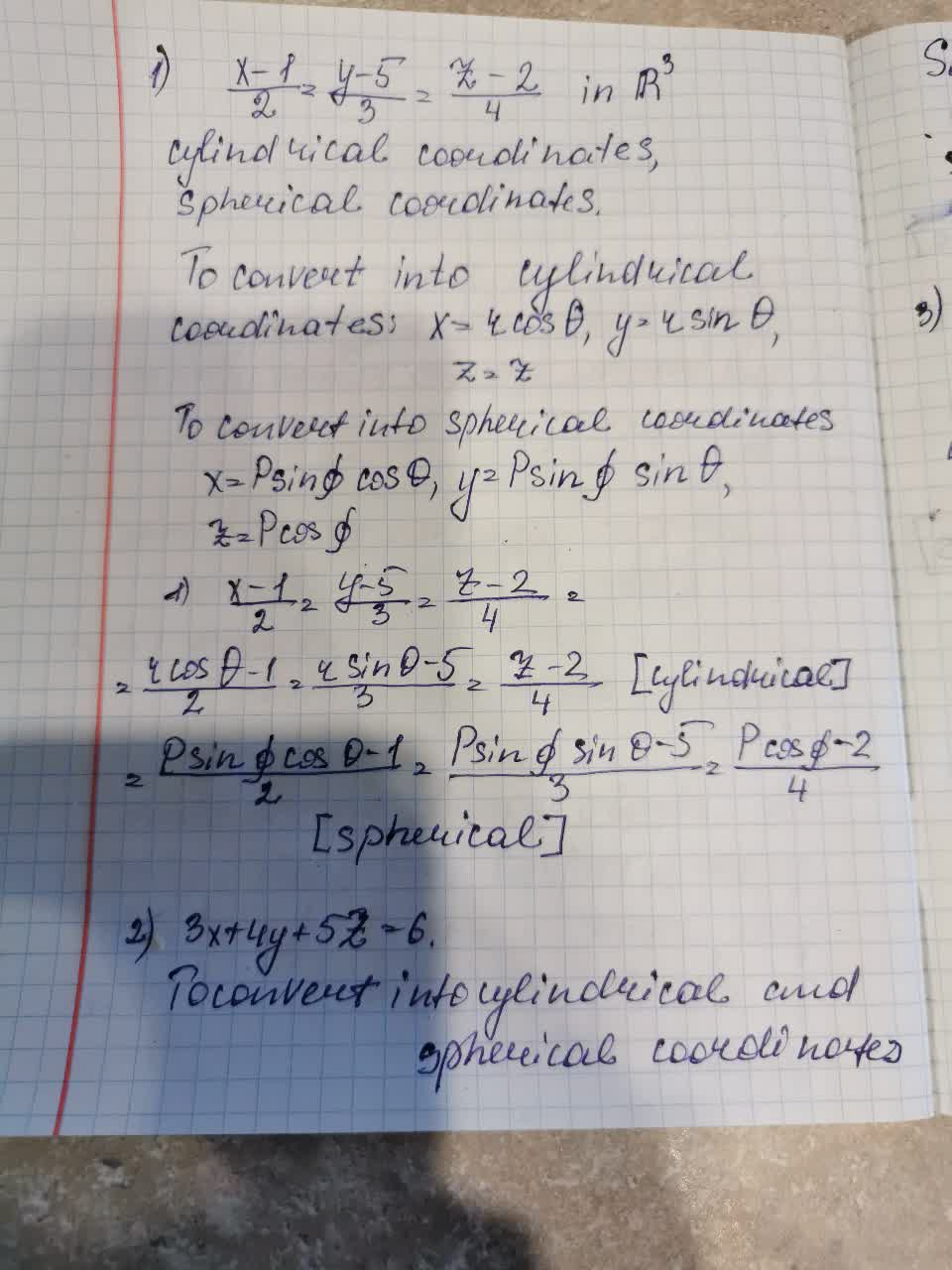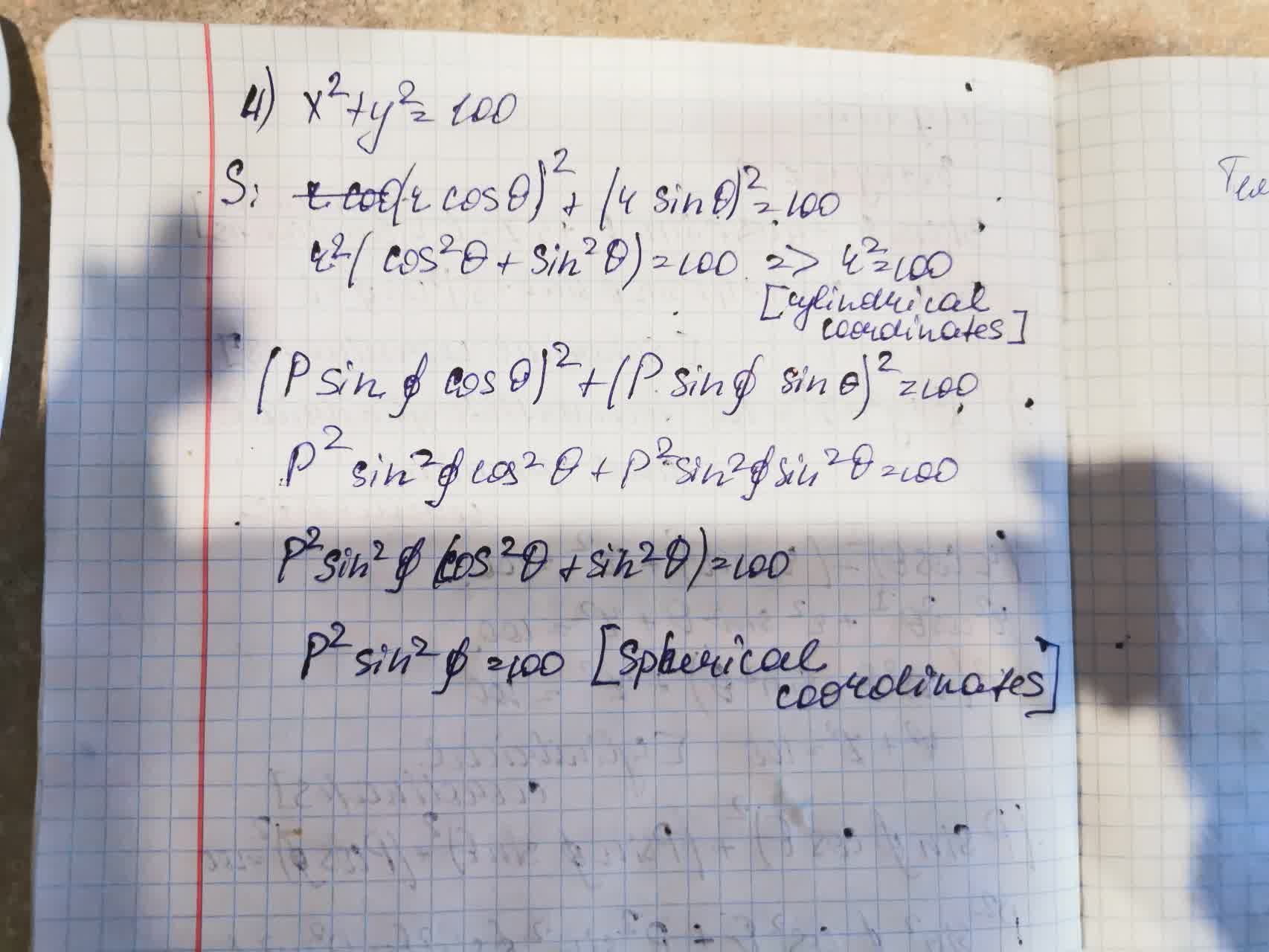# Write an equations in indicated coordinate system. Also write a parametric equation and a vector equation for each of the following equations.1) frac{x-1}{2}=frac{y-5}{3}=frac{z-2}{4}Tolnaio 2021-09-10 Answered

Write an equations in indicated coordinate system. Also write a parametric equation and a vector equation for each of the following equations.

1) $\frac{x-1}{2}=\frac{y-5}{3}=\frac{z-2}{4}$ cylindrical coordinates, sherical coordinates

2) $3x+4y+5z=6$ cylindrical coordinates, sherical coordinates

3) ${x}^{2}+{y}^{2}+{z}^{2}=100$ cylindrical coordinates, sherical coordinates

4) ${x}^{2}+{y}^{2}=100$ cylindrical coordinates, sherical coordinates

You can still ask an expert for help

• Questions are typically answered in as fast as 30 minutes

Solve your problem for the price of one coffee

• Math expert for every subject
• Pay only if we can solve itNicole Conner

Equations in indicated coordinate system is